# HDF5 files in Python

HDF5 file stands for Hierarchical Data Format 5. It is an open-source file which comes in handy to store large amount of data. As the name suggests, it stores data in a hierarchical structure within a single file. So if we want to quickly access a particular part of the file rather than the whole file, we can easily do that using HDF5. This functionality is not seen in normal text files hence HDF5 is becoming seemingly popular in fact of being a new concept. To use HDF5, numpy needs to be imported. One important feature is that it can attach metaset to every data in the file thus provides powerful searching and accessing. Let’s get started with installing HDF5 to the computer.

To install HDF5, type this in your terminal:

`pip install h5py`

We will use a special tool called HDF5 Viewer to view these files graphically and to work on them. To install HDF5 Viewer, type this code :

`pip install h5pyViewer`

As HDF5 works on numpy, we would need numpy installed in our machine too.

`python -m pip install numpy`

After all the installations are done, let’s see how can we write into an HDF5 file.

Note: Working with HDF5 requires basic understanding of `numpy` and its attributes, so one must be familiar with `numpy` in order to understand the codes following in this article. To know more about `numpy` click here.

We will create a file and save a random array of numpy in it:

 `# Python program to demonstrate ` `# HDF5 file ` ` `  `import` `numpy as np ` `import` `h5py ` ` `  `# initializing a random numpy array ` `arr ``=` `np.random.randn(``1000``) ` ` `  `# creating a file ` `with h5py.``File``(``'test.hdf5'``, ``'w'``) as f:  ` `    ``dset ``=` `f.create_dataset(``"default"``, data ``=` `arr) `

Output: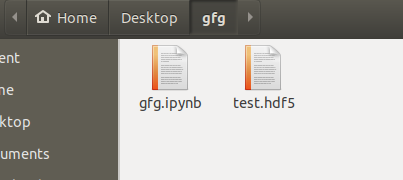In the above code, we first import the modules which were installed previously. Then we initialize the variable `arr` to a random array of `numpy` ranging till 1000.
Hence, we can say that this array consists of a large number of data. Next, we open the file as “write only” attribute. This means that if there isn’t any file with the name test.hdf5 then it will create one otherwise it will delete (overwrite) the content of the existing file. While opening the file, we used `with` instead of `open` as it has an upper-hand when compared to `open()` method. We don’t need to close a file if we open it using `with` Finally, we use the `.create_dataset()` to set the varibale dset to the array which was created earlier.

We will now read the file which we wrote above:

 `# open the file as 'f' ` `with h5py.``File``(``'test.hdf5'``, ``'r'``) as f:  ` `    ``data ``=` `f[``'default'``] ` `     `  `    ``# get the minimum value ` `    ``print``(``min``(data))  ` `     `  `    ``# get the maximum value ` `    ``print``(``max``(data)) ` `     `  `    ``# get the values ranging from index 0 to 15 ` `    ``print``(data[:``15``]) ` `    `

Output: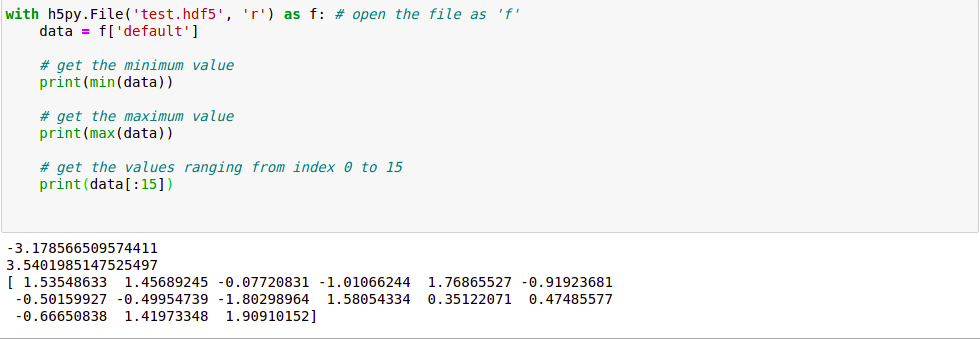Here, we open the file again but this time we open it by “read-only” attribute so that no changes can be made to the file. We set the variable `data` to the data we stored in the previous file. Let’s look at the output:

It may seem that there’s nothing new. It is just an array and we are printing out the numbers just as in an array. But, the variable `data` is not an array. It’s actually very different than array. It’s a dataset. Rather than storing data in the RAM, it saves it in hard-drive of the computer, thus maintaining a hierarchy of structures just like directories: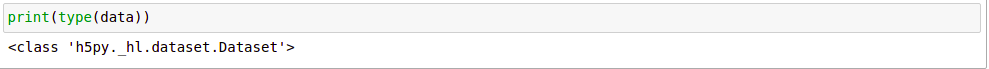When the below line is used

`data = f['default']`

in the previous code, we did not access the content of the file directly but created a pointer to point at our content. Let’s look at an advantage of this:

 `import` `numpy as np ` `import` `h5py ` ` `  ` `  `arr1 ``=` `np.random.randn(``10000``) ` `arr2 ``=` `np.random.randn(``10000``) ` ` `  `with h5py.``File``(``'test_read.hdf5'``, ``'w'``) as f: ` `    ``f.create_dataset(``'array_1'``, data ``=` `arr1) ` `    ``f.create_dataset(``'array_2'``, data ``=` `arr2) `

We created two datasets but the whole procedure is same as before. A file named “test_read.hdf5” is created using the “w” attribute and it contains two datasets (array1 and array2) of random numbers. Now suppose we want to read only a selective portion of array2. For example, we want to read that part of array2 corresponding to where values of array1 are greater than 1. If we were using the conventional text files instead of HDF5 files, it would be nearly impossible to achieve this. That’s exactly were we see the power of HDF5 files:

 `with h5py.``File``(``'test_read.hdf5'``, ``'r'``) as f: ` `    ``d1 ``=` `f[``'array_1'``] ` `    ``d2 ``=` `f[``'array_2'``] ` ` `  `    ``data ``=` `d2[d1[:]>``1``] `

We use the [:] to create a copy of the dataset d1 into the RAM. We did this because a dataset (the data in hard-drive) cannot be compared to the integers.
Output: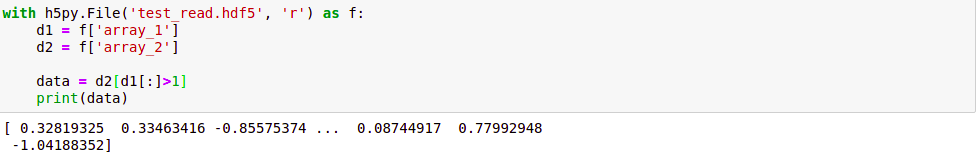So, we conclude that HDF5 files are our best tools when we are dealing with large files as it allows us selective reading and writing of files which otherwise would have consumed a lot of memory and time.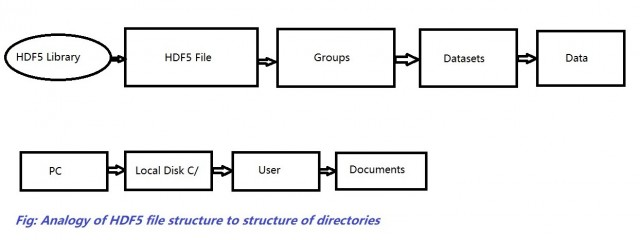My Personal Notes arrow_drop_upCheck out this Author's contributed articles.

If you like GeeksforGeeks and would like to contribute, you can also write an article using contribute.geeksforgeeks.org or mail your article to contribute@geeksforgeeks.org. See your article appearing on the GeeksforGeeks main page and help other Geeks.

Please Improve this article if you find anything incorrect by clicking on the "Improve Article" button below.

Article Tags :

Be the First to upvote.

Please write to us at contribute@geeksforgeeks.org to report any issue with the above content.